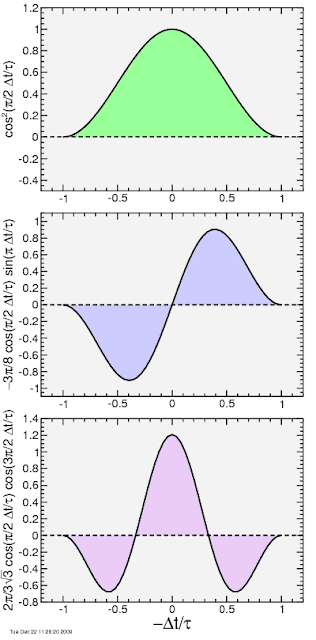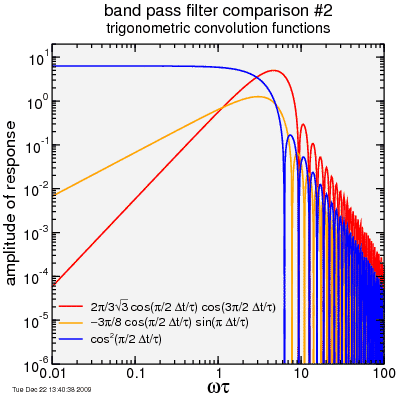## Tuesday, December 22, 2009

### Filters 2.0: frequency response

Now I'll go through the frequency response of my "new favorite" filters. These are all trigonometric functions, so no need for integration by parts, as long as I use a few trigonometric identities. I was even able to skip most of the expanding trigometric functions in terms of their complex exponential equivalents. I'll spare the details and cut to the chase.

Here's the filters again:In each case assume an input function:

f(t) = f0 sin ωt,

and where the output function is f*(t). In each case I'll explicitly include the time shift to make the filter causal, so I'll represent the output function delayed as f*(t + τ). This is the reason I've changed my definition of τ here: it now represents the half-width of the filter function; a more direct representation of the delay.

cos2(π/2 Δt/τ):

I analyzed this before, but with a slightly different form. The result:
f*(t + τ) = 2π f0 sin(ωt) sin(ωτ) / [ωτ (1 ‒ ω²τ²/π²)].

‒3π/2 cos(π/2 Δt/τ) sin(π Δt/τ):

Skipping a bunch of steps in which it's highly likely I made at least one mistake....

f*(t + τ) = f0 cos ωt (6 ωt / [(1 ‒ 4 ω²τ² / π²)(9 ‒ 4 ω²τ² / π²)]) cos ωτ.

And finally...

2π/3√3 cos(π/2 Δt/τ) cos(3π/2 Δt/τ):

Okay... hoping against hope I did proper bookkeeping here:
f*(t + τ) = f0 sin ωt ((4/√3) ωτ / [(1 ‒ ω²τ² / π²)(4 ‒ ω²τ² / π²)]) sin ωτ.

Let's plot these puppies. I may have a bug in the magnitude, but the shape of the curves should be correct:So the filters do basically what I wanted. All three attenuate high frequencies proportional to the cube of the frequency. At low frequencies is where the differ. The cosine square filter passes low frequencies. The cosine times sine filter lightly attenuates low frequencies. The cosine-cosine filter strongly cuts off low frequencies: a bit too much, honestly, for most of my applications.

One thing which is curious in that plot is why the red curve is shifted to higher frequencies. Well, it's obvious from the convolution shape that a frequency relatively aligned with the convolution function is going to have the maximal transmission amplitude. This frequency is clearly highest in the cos-cos filter. So if the focus is for a given delay (τ) to get the best suppression of high frequencies, the cos-sin filter is the better choice.

Anyway, enough of that. Hopefully that's all I need to say on filters. Unless I think of something else.